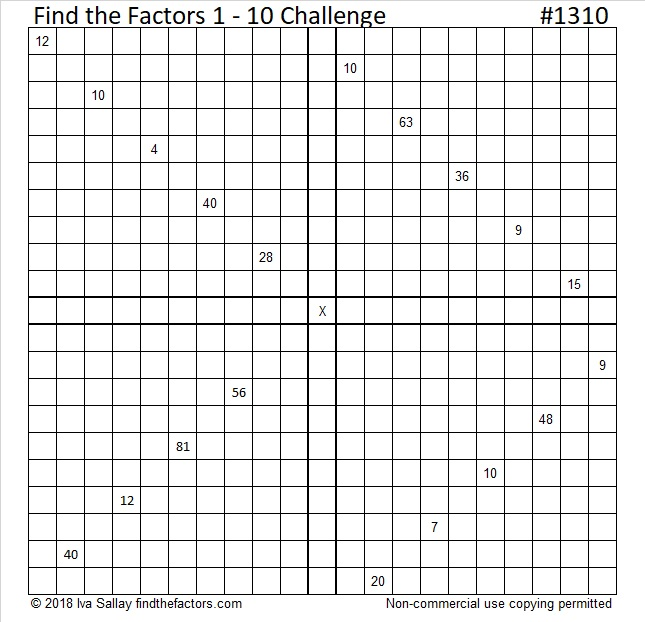# 1310 Happy Birthday to My Brother, Andy

Today is my brother’s birthday. He likes puzzles so I’ve made him a tough, challenging one. Still, he’ll probably figure it out in no time. Happy birthday, Andy!Print the puzzles or type the solution in this excel file: 10-factors-1302-1310

Like always, I’ll write what I’ve learned about a number. This time it’s 1310’s turn.

• 1310 is a composite number.
• Prime factorization: 1310 = 2 × 5 × 131
• The exponents in the prime factorization are 1, 1, and 1. Adding one to each and multiplying we get (1 + 1)(1 + 1)(1 + 1) = 2 × 2 × 2 = 8. Therefore 1310 has exactly 8 factors.
• Factors of 1310: 1, 2, 5, 10, 131, 262, 655, 1310
• Factor pairs: 1310 = 1 × 1310, 2 × 655, 5 × 262, or 10 × 131
• 1310 has no square factors that allow its square root to be simplified. √1310 ≈ 36.193921310 is the hypotenuse of a Pythagorean triple:
786-1048-1310 which is (3-4-5) times 262

As shown in their factor trees below, 1308, 1309, 1310, and 1311 each have three distinct prime numbers in their prime factorizations. They are the smallest set of four consecutive numbers with the same number of prime factors. 1309, 1310, and 1311 are also the smallest three consecutive numbers that have exactly the same number of factors and factor pairs. Thank you Stetson.edu for alerting me to those facts.